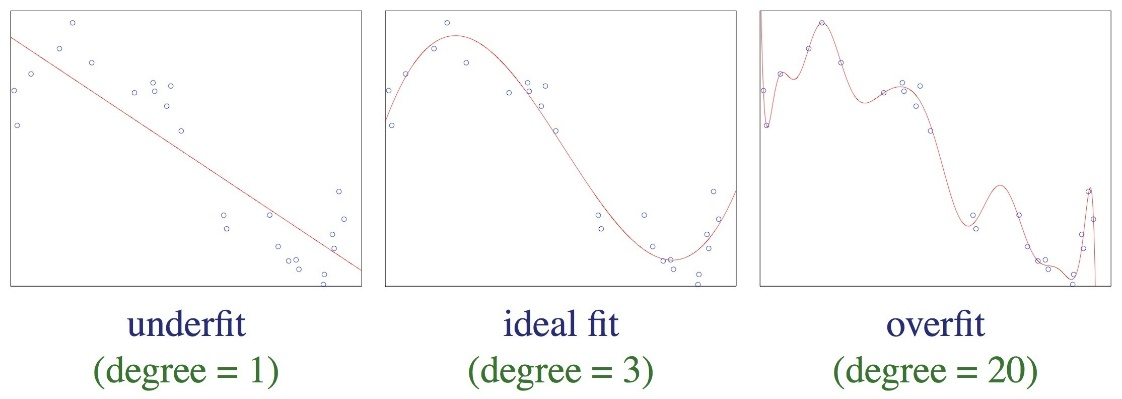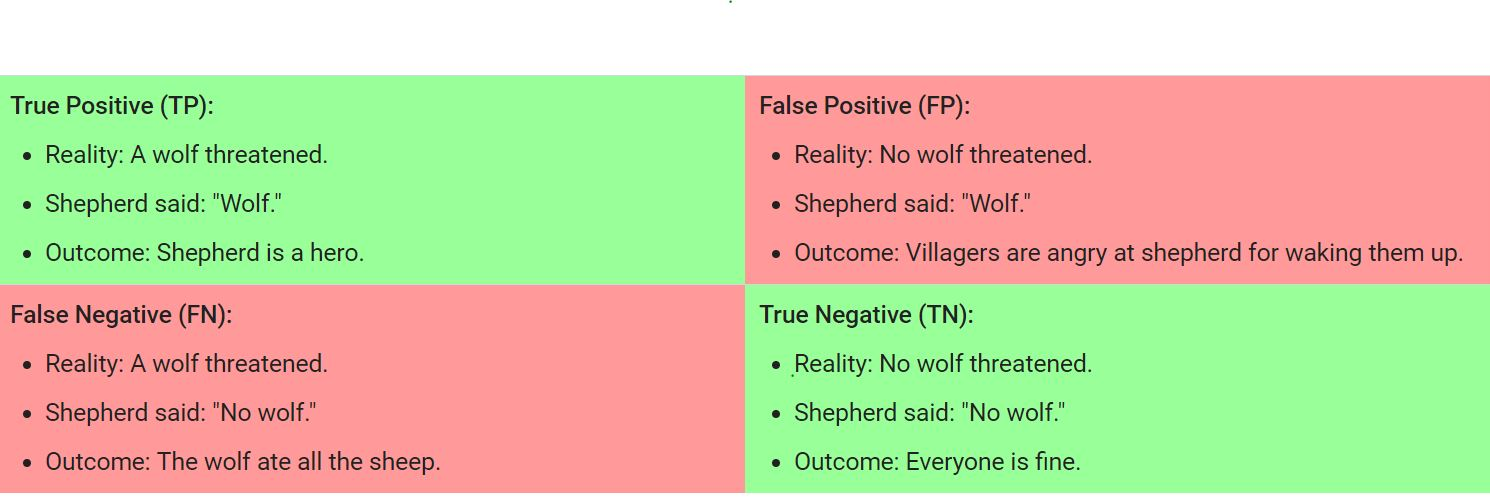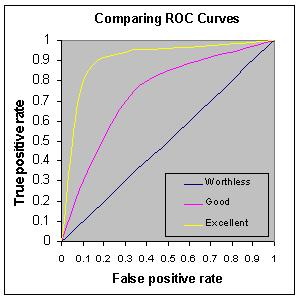# Machine Learning For Beginners 1: Must Know Terminologies

Data model: Data model involves providing an ML algorithm (that is, the learning algorithm) with training data to learn from. The term ML model refers to the model artifact that is created by the training process.

Data Leakage: Dictionary meaning of leakage is “deliberate disclosure of confidential information”. So, data leakage means leaking some data to your training model which can lead to over-fitting. e.g.

• Including feature as a label in model training
• Including test data in training data
• Distorting information from samples outside of the scope of the model’s intended use.
• Include Information from data samples outside of the scope of the algorithm’s intended use. Details ref1, ref2,ref3, ref4

Features: Features are the variables found in the given problem set that can strongly/sufficiently help us build an accurate predictive model.

Data Label vs Feature: Feature is input; label is output.

Cross-validation:  A mechanism for estimating how well a model will predict to new data by testing the model against one or more non-overlapping data subsets withheld from the training set.

Over-fitting vs Under-fitting vs ideal fit a model:Variance Vs Bias:

• error(X) = noise(X) + bias(X) + variance(X)
• bias(X): Learning wrong things. Away from accuracy. Under-fitting.
• variance(X): Learning random things.Over fitting.

Details: ref1

False Positive vs False Negative: A false positive is an outcome where the model incorrectly predicts the positive class. And a false negative is an outcome where the model incorrectly predicts the negative class.Details: ref1

Model parameter vs Model hyper-parameter: A model parameter is a configuration variable that is internal to the model and whose value can be estimated from data. Whereas, A model hyper-parameter is a configuration that is external to the model and whose value is usually set by the data scientist.

Details: ref1

AUC: Area Under the ROC Curve: Accuracy of model is measured by the area under the ROC curve. AUC ranges in value from 0 to 1. A model whose predictions are 100% wrong has an AUC of 0.0; one whose predictions are 100% correct has an AUC of 1.0.• 90-1 = excellent (A)
• .80-.90 = good (B)
• .70-.80 = fair (C)
• .60-.70 = poor (D)
• .50-.60 = fail (F)

AUC is desirable for the following two reasons:

• AUC is scale-invariant. It measures how well predictions are ranked, rather than their absolute values.
• AUC is classification-threshold-invariant. It measures the quality of the model’s predictions irrespective of what classification threshold is chosen.

More ref1 ,ref2